# User:Tohline/Appendix/Ramblings/RiemannB74C692

(Difference between revisions)
 Revision as of 10:36, 29 May 2020 (view source)Tohline (Talk | contribs) (→Another S-type Example b74c692)← Older edit Revision as of 13:30, 29 May 2020 (view source)Tohline (Talk | contribs) Newer edit → Line 1: Line 1: - + __FORCETOC__ =Another S-type Example b74c692= =Another S-type Example b74c692= Line 70: Line 70: The subscript "EFE" on Ω and λ means that the relevant frequency is given in units that have been adopted in [[[User:Tohline/Appendix/References#EFE|EFE]]], that is, in units of $~[\pi G\rho]^{1 / 2}$.  In Figure 1a, the solid purple circular marker (where the pair of purple lines cross) identifies the location of this model in the "c/a versus b/a" diagram that appears as Figure 2 on p. 902 of [http://adsabs.harvard.edu/abs/1965ApJ...142..890C S. Chandrasekhar (1965)]; essentially the same diagram appears in §49 (p. 147) of [[[User:Tohline/Appendix/References#EFE|EFE]]]. The subscript "EFE" on Ω and λ means that the relevant frequency is given in units that have been adopted in [[[User:Tohline/Appendix/References#EFE|EFE]]], that is, in units of $~[\pi G\rho]^{1 / 2}$.  In Figure 1a, the solid purple circular marker (where the pair of purple lines cross) identifies the location of this model in the "c/a versus b/a" diagram that appears as Figure 2 on p. 902 of [http://adsabs.harvard.edu/abs/1965ApJ...142..890C S. Chandrasekhar (1965)]; essentially the same diagram appears in §49 (p. 147) of [[[User:Tohline/Appendix/References#EFE|EFE]]]. + + ==COLLADA Model Files== + + +
+ b74c692DI.dae [''Direct'' Inertial Frame]    …     a COLLADA code containing nnnn lines of -formatted ASCII text +
+ +
+
+
+
+
+ + +
+ b74c692DRot.dae [''Direct'' Rotating Frame]    …     a COLLADA code containing nnnn lines of -formatted ASCII text +
+ +
+
+
+
+
+ + +
+ b74c692AI.dae [''Adjunct'' Inertial Frame]    …     a COLLADA code containing nnnn lines of -formatted ASCII text +
+ +
+
+
+
+
+ + +
+ b74c692ARot.dae [''Adjunct'' Rotating Frame]    …     a COLLADA code containing nnnn lines of -formatted ASCII text +
+ +
+
+
+
+

# Another S-type Example b74c692

This chapter is an extension of the chapter we have titled, "Riemann Meets COLLADA & Oculus Rift S." In that chapter we used as our first example of a Riemann S-type ellipsoid the model with parameters, (b/a, c/a) = (0.41, 0.385). Here we construct a model with parameters, (b/a, c/a) = (0.74, 0.692). Other closely related chapters are listed below under the heading, "See Also".

## Key Physical Parameters

The model that we have chosen to use in our second successful construction of a COLLADA-based, 3D and interactive animation has the following properties; this model has been selected from Table 2 of our accompanying discussion of Riemann S-type ellipsoids: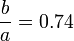$~\frac{b}{a} = 0.74$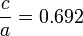$~\frac{c}{a} = 0.692$ Direct Adjoint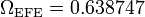$~\Omega_\mathrm{EFE} = 0.638747$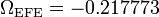$~\Omega_\mathrm{EFE} = - 0.217773$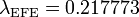$~\lambda_\mathrm{EFE} = 0.217773$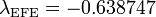$~\lambda_\mathrm{EFE} = - 0.638747$

The subscript "EFE" on Ω and λ means that the relevant frequency is given in units that have been adopted in [EFE], that is, in units of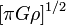$~[\pi G\rho]^{1 / 2}$. In Figure 1a, the solid purple circular marker (where the pair of purple lines cross) identifies the location of this model in the "c/a versus b/a" diagram that appears as Figure 2 on p. 902 of S. Chandrasekhar (1965); essentially the same diagram appears in §49 (p. 147) of [EFE].

b74c692DI.dae [Direct Inertial Frame]    …     a COLLADA code containing nnnn lines of <xml>-formatted ASCII text

<?xml version="1.0" encoding="UTF-8" standalone="no" ?>


b74c692DRot.dae [Direct Rotating Frame]    …     a COLLADA code containing nnnn lines of <xml>-formatted ASCII text

<?xml version="1.0" encoding="UTF-8" standalone="no" ?>


b74c692AI.dae [Adjunct Inertial Frame]    …     a COLLADA code containing nnnn lines of <xml>-formatted ASCII text

<?xml version="1.0" encoding="UTF-8" standalone="no" ?>


b74c692ARot.dae [Adjunct Rotating Frame]    …     a COLLADA code containing nnnn lines of <xml>-formatted ASCII text

<?xml version="1.0" encoding="UTF-8" standalone="no" ?>